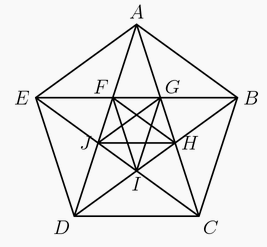###### back to index | new

With all angles measured in degrees, the product $\displaystyle\prod_{k=1}^{45} csc^2(2k-1)^\circ=m^n$, where $m$ and $n$ are integers greater than 1. Find $m+n$.

Define the sequence $a_1, a_2, a_3, \ldots$ by $a_n = \sum\limits_{k=1}^n \sin{k}$, where $k$ represents radian measure. Find the index of the $100^{th}$ term for which $a_n < 0$.

Let $f(x)=(x^2+3x+2)^{cos(\pi x)}$. Find the sum of all positive integers $n$ for which $\left |\sum_{k=1}^nlog_{10}f(k)\right|=1.$

Suppose that the angles of $\triangle ABC$ satisfy $cos(3A)+cos(3B)+cos(3C)=1.$ Two sides of the triangle have lengths $10$ and $13$. There is a positive integer $m$ so that the maximum possible length for the remaining side of $\triangle ABC$ is $\sqrt{m}$. Find $m$.

The domain of the function $f(x) = \arcsin(\log_{m}(nx))$ is a closed interval of length $\frac{1}{2013}$ , where $m$ and $n$ are positive integers and $m>1$. Find the remainder when the smallest possible sum $m+n$ is divided by 1000.

For $\pi \le \theta < 2\pi$, let\begin{align*} P &= \frac12\cos\theta - \frac14\sin 2\theta - \frac18\cos 3\theta + \frac{1}{16}\sin 4\theta + \frac{1}{32} \cos 5\theta - \frac{1}{64} \sin 6\theta - \frac{1}{128} \cos 7\theta + \cdots \end{align*} and \begin{align*} Q &= 1 - \frac12\sin\theta -\frac14\cos 2\theta + \frac18 \sin 3\theta + \frac{1}{16}\cos 4\theta - \frac{1}{32}\sin 5\theta - \frac{1}{64}\cos 6\theta +\frac{1}{128}\sin 7\theta + \cdots \end{align*} so that $\frac{P}{Q} = \frac{2\sqrt2}{7}$. Then $\sin\theta = -\frac{m}{n}$ where $m$ and $n$ are relatively prime positive integers. Find $m+n$.

Let $A,B,C$ be angles of an acute triangle with $$\cos^2 A + \cos^2 B + 2 \sin A \sin B \cos C = \frac{15}{8}$$ and $$\cos^2 B + \cos^2 C + 2 \sin B \sin C \cos A = \frac{14}{9}$$ There are positive integers $p$, $q$, $r$, and $s$ for which $\cos^2 C + \cos^2 A + 2 \sin C \sin A \cos B = \frac{p-q\sqrt{r}}{s},$ where $p+q$ and $s$ are relatively prime and $r$ is not divisible by the square of any prime. Find $p+q+r+s$.

Let $x$ and $y$ be real numbers such that $\frac{\sin x}{\sin y} = 3$ and $\frac{\cos x}{\cos y} = \frac12$. The value of $\frac{\sin 2x}{\sin 2y} + \frac{\cos 2x}{\cos 2y}$ can be expressed in the form $\frac pq$, where $p$ and $q$ are relatively prime positive integers. Find $p+q$.

Suppose $x$ is in the interval $[0, \frac{\pi}{2}]$ and $\log_{24\sin x} (24\cos x)=\frac{3}{2}$. Find $24\cot^2 x$.

Rational numbers $a$ and $b$ are chosen at random among all rational numbers in the interval $[0,2)$ that can be written as fractions $\frac{n}{d}$ where $n$ and $d$ are integers with $1 \le d \le 5$. What is the probability that $(\text{cos}(a\pi)+i\text{sin}(b\pi))^4$ is a real number?

Two circles intersect at points $A$ and $B$. The minor arcs $AB$ measure $30^\circ$ on one circle and $60^\circ$ on the other circle. What is the ratio of the area of the larger circle to the area of the smaller circle?

In $\triangle BAC$, $\angle BAC=40^\circ$, $AB=10$, and $AC=6$. Points $D$ and $E$ lie on $\overline{AB}$ and $\overline{AC}$ respectively. What is the minimum possible value of $BE+DE+CD$?

Find the sum of all the positive solutions of $2\cos2x \left(\cos2x - \cos{\left( \frac{2014\pi^2}{x} \right) } \right) = \cos4x - 1$

The angles in a particular triangle are in arithmetic progression, and the side lengths are $4,5,x$. The sum of the possible values of x equals $a+\sqrt{b}+\sqrt{c}$ where $a, b$, and $c$ are positive integers. What is $a+b+c$?

For $135^\circ < x < 180^\circ$, points $P=(\cos x, \cos^2 x), Q=(\cot x, \cot^2 x), R=(\sin x, \sin^2 x)$ and $S =(\tan x, \tan^2 x)$ are the vertices of a trapezoid. What is $\sin(2x)$?

Let $f(x) = \log_{10} \left(\sin(\pi x) \cdot \sin(2 \pi x) \cdot \sin (3 \pi x) \cdots \sin(8 \pi x)\right)$. The intersection of the domain of $f(x)$ with the interval $[0,1]$ is a union of $n$ disjoint open intervals. What is $n$?

In $\triangle ABC$, $\cos(2A-B)+\sin(A+B)=2$ and $AB=4$. What is $BC$?

A geometric sequence $(a_n)$ has $a_1=\sin x$, $a_2=\cos x$, and $a_3= \tan x$ for some real number $x$. For what value of $n$ does $a_n=1+\cos x$?

For how many values of $x$ in $[0,\pi]$ is $\sin^{ - 1}(\sin 6x) = \cos^{ - 1}(\cos x)$? Note: The functions $\sin^{ - 1} = \arcsin$ and $\cos^{ - 1} = \arccos$ denote inverse trigonometric functions.

Suppose that $\sin a + \sin b = \sqrt{\frac{5}{3}}$ and $\cos a + \cos b = 1$. What is $\cos (a - b)$?

For each integer $n>1$, let $F(n)$ be the number of solutions to the equation $\sin{x}=\sin{(nx)}$ on the interval $[0,\pi]$. What is $\displaystyle\sum_{n=2}^{2007} F(n)$?

Suppose $\cos x=0$ and $\cos (x+z)=\frac{1}{2}$. What is the smallest possible positive value of $z$?

Let $S$ be the set of all point $(x,y)$ in the coordinate plane such that $0 \le x \le \frac{\pi}{2}$ and $0 \le y \le \frac{\pi}{2}$. What is the area of the subset of $S$ for which $\sin^2x-\sin x \sin y + \sin^2y \le \frac34?$

In the figure shown below, $ABCDE$ is a regular pentagon and $AG=1$. What is $FG + JH + CD$?Rectangle $ABCD$ has $AB = 6$ and $BC = 3$. Point $M$ is chosen on side $AB$ so that $\angle AMD = \angle CMD$. What is the degree measure of $\angle AMD$?